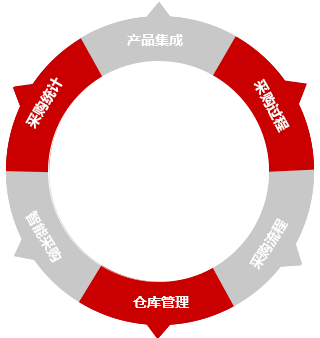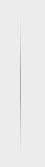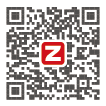PURCHASE SOLUTION• 核心功能
• 供应商
• 计划
• 询价
• 预购
• 采购
• 审批
• 到货
• 质检
• 仓库
• 应用工具
• 条形码
• 二维码
• 序列号
• 批号批次
• 保质期
• 扫描枪
• 采集器
• 盘点机
• 打印机

• 采购全程可视
• 仓库自由调度
• 产品集中管控
• 采购全面协同
• 采购自动高效
• 采购统计分析
• 供应商
• 询价
• 预购
• 采购
• 审批
• 到货
• 质检
• 入库

• 直接出入库
• 调拨出入库
• 盘点出入库
• 采购入库
• 退货入库
• 销售出库
• 借货出库
• 自由调拨

• 名称
• 编号
• 分类
• 型号
• MRP
• 图片
• 价格
• 单位

• 供应商
• 询价
• 预购
• 采购
• 审批
• 到货
• 质检
• 入库

• 无缝衔接
• 自动流转
• 一键跟踪
• 多维检索
• 明细导入
• 合并采购
• 到货提醒
• 模板套打

• 在线分析
• 离线分析
• 多维报表
• 数据关联
• 动态图
• 柱状图
• 折线图
• 饼状图

400-650-8060

+ 智邦一体化ERP
+ 集团及分公司

+ 关于我们
+ 联系我们

400热线：400-650-8060

+ 反馈中心扫一扫关注

400-650-8060

X

X